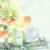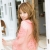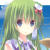# 可爱的 Python : Python中函数式编程，第一部分已翻译 100%

enixyu 投递于 2013/03/02 15:01 (共 12 段, 翻译完成于 03-04)

#### 参与翻译 (3人) : fbm, showme, Dinfer

D

• 函数具有首要地位 (对象)。也就是说，能对“数据”做什么事，就要能对函数本身做到那些事（比如将函数作为参数传递给另外一个函数）。
• 将递归作为主要的控制结构。在有些函数式语言中，都不存在其它的“循环”结构。
• 列表处理作为一个重点（例如，Lisp语言的名字）。列表往往是通过对子列表进行递归取代了循环。
• “纯”函数式语言会完全避免副作用。这么做就完全弃绝了命令式语言中几乎无处不在的这种做法：将第一个值赋给一个变量之后为了跟踪程序的运行状态，接着又将另外一个值赋给同一个变量。
• 函数式编程不是不鼓励就是完全禁止使用语句，而是通过对表达式(换句话说，就是函数加上参数）求值（evaluation of expressions）完成任务. 在最纯粹的情形下，一个程序就是一个表达式（再加上辅助性的定义）
• 函数式编程中最关心的是要对什么进行计算，而不是要怎么来进行计算。
• 在很多函数式编程语言中都会用到“高阶”（higher order）函数 (换句话说，高阶函数就是对对函数进行运算的函数进行运算的函数）。

## Python内在的函数式功能

Python中函数式编程的基本要素包括functionsmap()、reduce()、filter()和lambda算子（operator）。 在Python 1.x中，apply()函数也可以非常方便地拿来将一个函数的列表返回值直接用于另外一个函数。Python 2.0为此提供了一个改进后的语法。可能有点让人惊奇，使用如此之少的函数（以及基本的算子）几乎就足以写出任何Python程序了；更加特别的是，几乎用不着什么执行流程控制语句。

## 剔除流程控制语句

```# Normal statement-based flow control
if <cond1>:   func1()
elif <cond2>: func2()
else:         func3()

# Equivalent "short circuit" expression
(<cond1> and func1()) or (<cond2> and func2()) or (func3())

# Example "short circuit" expression
>>> x = 3
>>> def pr(s): return s
>>> (x==1 and pr('one')) or (x==2 and pr('two')) or (pr('other'))
'other'
>>> x = 2
>>> (x==1 and pr('one')) or (x==2 and pr('two')) or (pr('other'))
'two'```

Python中短路的Lambda

```>>> pr = lambda s:s
>>> namenum = lambda x: (x==1 and pr("one")) \
....                  or (x==2 and pr("two")) \
....                  or (pr("other"))
'one'
'two'
'other'```

• map()针对指定给它的一个或多个列表中每一项对应的内容，执行一次作为参数传递给它的那个函数 ，最后返回一个结果列表。
• reduce()针对每个后继项以及最后结果的累积结果，执行一次作为参数传递给它的那个函数；例如，reduce(lambda n,m:n*m, range(1,10))是求"10的阶乘"的意思（换言之，将每一项和前面所得的乘积进行相乘）
• filter()使用那个作为参数传递给它的函数，对一个列表中的所有项进行”求值“，返回一个由所有能够通过那个函数测试的项组成的经过遴选后的列表。

## Python中的函数式循环

```for e in lst:  func(e)      # statement-based loop
map(func,lst)           # map()-based loop```

Map-based 动作序列

```# let's create an execution utility function
do_it = lambda f: f()

# let f1, f2, f3 (etc) be functions that perform actions

map(do_it, [f1,f2,f3])   # map()-based action sequence```

Python中的函数式"while"循环

```# statement-based while loop
while <cond>:
<pre-suite>
if <break_condition>:
break
else:
<suite>

# FP-style recursive while loop
def while_block():
<pre-suite>
if <break_condition>:
return 1
else:
<suite>
return 0

while_FP = lambda: (<cond> and while_block()) or while_FP()
while_FP()```

Python中'echo'循环

```# imperative version of "echo()"
def echo_IMP():
while 1:
x = raw_input("IMP -- ")
if x == 'quit':
break
else
print x
echo_IMP()

# utility function for "identity with side-effect"
print x
return x

# FP version of "echo()"
echo_FP = lambda: monadic_print(raw_input("FP -- "))=='quit' or echo_FP()
echo_FP()
```

## 避免副作用

```# Nested loop procedural style for finding big products
xs = (1,2,3,4)
ys = (10,15,3,22)
bigmuls = []
# ...more stuff...
for x in xs:
for y in ys:
# ...more stuff...
if x*y > 25:
bigmuls.append((x,y))
# ...more stuff...
# ...more stuff...
print bigmuls```

```bigmuls = lambda xs,ys: filter(lambda (x,y):x*y > 25, combine(xs,ys))
combine = lambda xs,ys: map(None, xs*len(ys), dupelms(ys,len(xs)))
dupelms = lambda lst,n: reduce(lambda s,t:s+t, map(lambda l,n=n: [l]*n, lst))
print bigmuls((1,2,3,4),(10,15,3,22))```

### 评论(16)